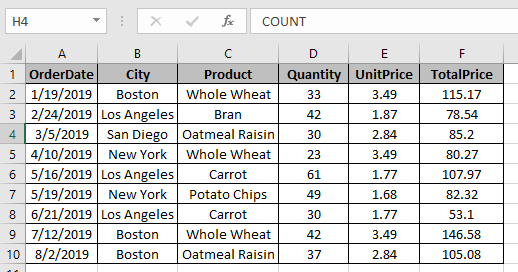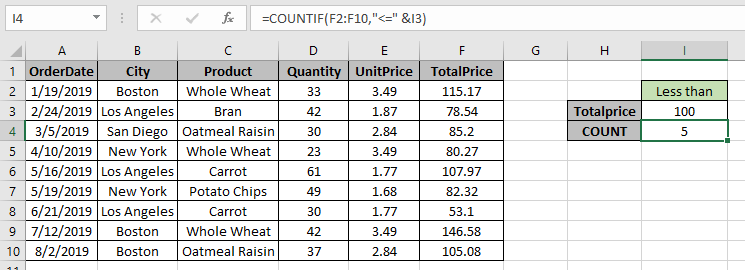# How to Count cells if less than value in Excel

In this article, we will learn about how to count cells if value is less than a particular values using COUNTIF in Excel.

In simple words, while working with long data sheet. Sometimes we need to find the count thee number of values if only the value in the cell is less than a particular value or say
we need to count values where data value of the corresponding values is less than a given value.

COUNTIF function returns the sum of range if date is greater than the given date.
Syntax:

=COUNTIF(range, "<" & value)

range : Set of Values
& : operator used to concatenate to other operator.
Let’s understand this function using it in an example.Here we need to find the TotalPrice COUNT if the TotalPrice is less than 100.
So we will use the formula to get the amount

=COUNTIF(F2:F10,"<" &I3)

"<" & I3 : criteria, less than the value in I3 which is 100.
F2:F10 : rangeThere are 5 cells where Value of TotalPrice is less than 100.

Hope you understood how to count cells if less than a given value in Excel. Explore more articles on Excel COUNTIF functions here. Please feel free to state your query or feedback for the above article.

Related articles:

How to count cells between values

How to COUNTIFS Two Criteria Match in Excel

How to do Running Count of Occurance in Excel

Popular Articles :

50 Excel Shortcut to Increase Your Productivity : Get faster at your task. These 50 shortcuts will make you work even faster on Excel.

How to use the VLOOKUP Function in Excel : This is one of the most used and popular functions of excel that is used to lookup value from different ranges and sheets.

How to use the COUNTIF function in Excel : Count values with conditions using this amazing function. You don't need to filter your data to count specific values. Countif function is essential to prepare your dashboard.

How to use the SUMIF Function in Excel : This is another dashboard essential function. This helps you sum up values on specific conditions.

Terms and Conditions of use

The applications/code on this site are distributed as is and without warranties or liability. In no event shall the owner of the copyrights, or the authors of the applications/code be liable for any loss of profit, any problems or any damage resulting from the use or evaluation of the applications/code.